# 640.1 Pavement Drainage

## 640.1.1 General

The term pavement drainage refers to the removal of storm water from paved areas, such as traffic lanes, paved shoulders and paved medians. Only those situations where storm water must be carried on the pavement surface by means of roadway gutters are considered in pavement drainage. The mathematical models, procedures and design criteria presented herein are to be used in the analysis of gutter flow and in the selection and spacing of storm water inlets. The design of storm sewer systems is discussed in Storm Sewers.

## 640.1.2 Design Controls

The sizing and spacing of storm water inlets are subject to certain design controls which will define the spacing required for any given situation. These criteria define the frequency of the design storm, the allowable gutter spread, the allowable depth of gutter flow and the required inlet intercept.

### 640.1.2.1 Design Frequency

EPG 640.1.2.1 applies to roadway design frequency only. For details regarding design frequency on bridges, see EPG 751.10.3 Bridge Deck Drainage – Slab Drains.

For major roads, choose a storm frequency in the range of 10 to 50 years. For minor roads, choose a storm frequency in the range of 10 to 25 years.

Typical Locations - The higher frequency (i.e., 10-year) criteria will apply to design of most inlets, including those inlets located at low points in grade.

Critical Locations - The lower frequencies (i.e., 50-year) should be used for design of critical low points, such as underpasses or high traffic volume "at grade" intersections.

Non-Typical Locations – For locations with non-typical features such as super elevation transitions, entrances with slotted drains and non-standard shoulders, a frequency in between the high and low frequencies may be appropriate.

Consideration is also given to AADT and importance of route when choosing a design storm frequency.

Major Typical 10-year
Non-Typical 10-year to 50-year
Critical 50-year
Minor Typical 10-year
Non-Typical 10-year to 25-year
Critical 25-year

The duration of the design storm is set equal to the time of concentration of the drainage area or five minutes, whichever is greater. The peak rates of runoff produced by the design storm is computed by The Rational Method.

EPG 640.1.2.2 applies to roadway gutter spread only. For details regarding gutter spread on bridges, see EPG 751.10.3 Bridge Deck Drainage – Slab Drains.

Spread is defined as the width of gutter flow, measured laterally from the face of the curb. Allowable spread depends on the classification of the roadway and importance of route to the community as determined by the Core Team. Gutter spreads shown below are maximum widths, other design factors including ADA requirements should be considered when determining the allowable gutter spread.

Interstates

For all interstates, gutter spread shall not encroach upon the traveled lanes. Gutter spread shall be limited to the shoulder and shall not exceed 12 ft. during the design runoff event.

For all major roads with a posted speed of 45 mph or greater, gutter spread shall not encroach upon the traveled lanes. Gutter spread shall be limited to the shoulder and/or parking lane and shall not exceed 12 ft. during the design runoff event.

For major roads with a posted speed of less than 45 mph:

a) For 2-lane facilities or when the cross slope only extends across 1 lane, gutter spread may encroach up to 3 ft. into the driving lane.
b) On multilane facilities, when 2 or more lanes in one direction have their cross slopes running in a single direction, the gutter spread may encroach up to ½ of the lane nearest the gutter section for those lanes.

For minor roads with a posted speed of 45 mph or greater:

a) For 2-lane facilities or when the cross slope only extends across 1 lane, gutter spread may encroach up to 3 ft. into the driving lane.
b) On multilane facilities, when 2 or more lanes in one direction have their cross slopes running in a single direction, the gutter spread may encroach up to ½ of the lane nearest the gutter section for those lanes.

For minor roads with a posted speed of less than 45 mph, a gutter spread of up to ½ lane may be used.

Single Lanes

For one lane facilities, such as ramps, a minimum of 8’ of the traveled lane shall remain unflooded.

Interstate All Up to the shoulder width, with a 12’ max.
Major ≥ 45 mph Up to the shoulder and/or park lane width, with a 12’ max.
2-Lane roads < 45 mph Shoulder + 3 ft.
*Multilane roads < 45 mph Shoulder + ½ lane
Minor 2-Lane roads ≥ 45 mph Shoulder + 3 ft.
*Multilane roads > 45 mph Shoulder + ½ lane
< 45 mph Shoulder + ½ lane
Single Lane All Minimum of 8 ft. unflooded
* 2 or more lanes in one direction that have their cross slope in the same direction.

### 640.1.2.3 Depth of Gutter Flow

The allowable depth of gutter flow is a function of the height of curb and is given in the table below.

Allowable Depth of Gutter Flow
Height of Curb Maximum Depth of Flow
(in.) (mm) (ft.) (mm)
3 75 0.200 60
4 100 0.267 80
6 150 0.400 120
8 200 0.450 135

In no case should the depth of gutter flow exceed the values given in the above table during the design runoff event.

### 640.1.2.4 Intercept on Continuous Grade

Except in special cases, no attempt is made to intercept all water arriving at inlets located on continuous grades. In general these inlets are designed to intercept no more than 70% of the total design gutter flow.

One exception to the above rule is the case of bridge approaches. Where a bridge is located on a continuous grade, approaching storm water is not allowed to flow onto the bridge deck. A drop inlet or series of drop inlets as needed are used to intercept all gutter flow at the upstream end of the bridge. These inlets should be located outside of the bridge approach slab if possible.

Water flowing across an intersection may cause a certain amount of danger to cross traffic and should be limited. Therefore, inlets placed at the upstream side of intersections are sized so that no more than 2.0 ft3/s (0.05 m3/s) is allowed to flow into the intersection during the design event.

Where the pavement transitions from crown to superelevated sections or from superelevated to crown sections all storm water is intercepted to prevent cross flow over the pavement. Similarly, all storm water at the downstream end of curbed ramps is intercepted, when this water would flow across the ramp or main roadway pavement.

### 640.1.2.5 Intercept at Low Points

Inlets located at low points in grade are designed to intercept all water arriving at the inlet. The allowable depth of ponding is controlled by the criteria of maximum depth at the curb or maximum spread, as previously discussed. The most restrictive of these criteria control.

## 640.1.3 Flow of Water in Gutters

The flow of water in shallow triangular channels such as roadway gutters may be analyzed by a special form of Manning's equation.

### 640.1.3.1 Triangular Gutter Sections

For triangular gutter sections, the following form of Manning's equation may be used: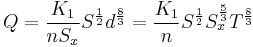$Q=\frac{K_1}{nS_x}S^{\frac{1}{2}}d^{\frac{8}{3}}=\frac{K_1}{n}S^{\frac{1}{2}}S_x^{\frac{5}{3}}T^{\frac{8}{3}}$
where:
Q = total gutter flow rate, ft3/s (m3/s)
K1 = constant, 0.56 - ENGLISH (0.376 - METRIC)
n = Manning's roughness coefficient (0.016 for gutters and pavement)
Sx = Pavement and gutter cross-slope, ft/ft (m/m)
S = Longitudinal pavement slope (grade), ft/ft (m/m)
d = depth of flow at face of curb, ft. (m)

Normally, the gutter flow rate and gutter cross-section are known and the depth of flow is desired. In this case, the following form of the above Equation may be used.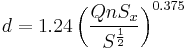$d=1.24\left(\frac{QnS_x}{S^{\frac{1}{2}}}\right)^{0.375}$
where: C = constant, 1.24 - ENGLISH (1.44 - METRIC)
The spread in a triangular section can be calculated using the equation: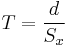$T=\frac{d}{S_x}\,$
where:
T = spread (width of gutter flow), ft. (m)
d = depth of flow at face of curb, ft. (m)
Sx = Pavement and gutter cross-slope, ft/ft (m/m)
The average velocity in a gutter section is calculated using the equation: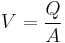$V=\frac{Q}{A}\,$
Where:
V = average velocity, ft/s (m/s)
Q = discharge, ft3/s (m3/s)
A = cross-sectional area of flow, ft2 (m2)
The area can be calculated using the equation: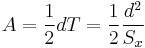$A=\frac{1}{2}dT=\frac{1}{2}\frac{d^2}{S_x}$
where:
A = cross-sectional area of flow, ft2 (m2)
Sx = Pavement cross-slope, ft/ft (m/m)
d = depth of flow at face of curb, ft. (m)

### 640.1.3.2 Composite Curb-and-Gutter Sections

For a Composite Curb-and-Gutter Section, where the gutter slope is different from the pavement cross-slope, the composite section is divided into triangular sub-sections. The above equation can then be applied to sub-triangles to determine the total flow in the composite section. The equation is applied to three separate triangles ABE, DCE, and DCF as shown on the Composite Curb-and-Gutter Section. The total flow is determined by adding the flows from triangles ABE and DCF and subtracting the flow from triangle DCE. The modified form of Manning's equation can be used to calculate flows for various complex shapes in this manner.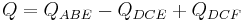$Q=Q_{ABE}-Q_{DCE}+Q_{DCF}\,$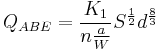$Q_{ABE}=\frac{K_1}{n\frac{a}{W}}S^\frac{1}{2}d^\frac{8}{3}$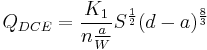$Q_{DCE}=\frac{K_1}{n\frac{a}{W}}S^\frac{1}{2}(d-a)^\frac{8}{3}$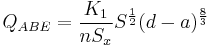$Q_{ABE}=\frac{K_1}{nS_x}S^\frac{1}{2}(d-a)^\frac{8}{3}$
where:
K1 = constant, 0.56 - ENGLISH (0.376 - METRIC)
n = Manning's roughness coefficient (0.016 for gutters and pavement)
a = gutter depression, ft. (m)
W = gutter width, ft. (m)
S = Longitudinal pavement slope (grade), ft/ft (m/m)
Sx = Pavement cross-slope, ft/ft (m/m)
d = depth of flow at face of curb, ft. (m)

For a composite section where the depth of flow is greater than the gutter depression, the spread can be calculated using the equation: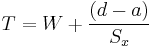$T=W+\frac{(d-a)}{S_x}$
where:
Sx = Pavement cross-slope, ft/ft (m/m)
d = depth of flow at face of curb, ft. (m)
a = gutter depression, ft. (m)
W = gutter width, ft. (m)

For a composite curb-and-gutter section where the depth of flow is greater than the gutter depression, the area can be calculated using the equation: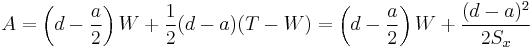$A=\left(d-\frac{a}{2}\right)W+\frac{1}{2}(d-a)(T-W)=\left(d-\frac{a}{2}\right)W+\frac{(d-a)^2}{2S_x}$
where:
A = cross-sectional area of flow, ft2 (m2)
Sx = Pavement cross-slope, ft/ft (m/m)
d = depth of flow at face of curb, ft. (m)
a = gutter depression, ft. (m)
W = gutter width, ft. (m)

## 640.1.4 Types of Storm Water Inlets

Two different types of precast storm water inlets are used in pavement drainage applications. They are grate inlets and curb opening inlets. Guidance for specifying and inspecting drop inlets and grates is in Precast Reinforced Concrete Manholes and Drop Inlets.

### 640.1.4.1 Grate Inlets (Precast Type A, B, C & D)

Standard curved vane grate inlets are 2 ft. (610 mm) long (measured in the direction of flow), and either 2 or 4 ft. (610 or 1219 mm) wide (measured perpendicular to the direction of flow). Only 2 ft. (610 mm) wide inlets may be used in curb and gutter sections; whereas 2 or 4 ft. widths may be used in conjunction with integral curb (triangular) sections. Two types of inlet grates are shown in the standard plans. The curved vane grate is used in roadway and shoulder applications. The parallel bar grate may be used only in areas outside the roadway and shoulders, such as in grassy medians or other unpaved areas. See Standard Plan details of inlet grates (Std. Plans 614.10 and 614.11) and gutter cross sections (Std. Plan 609.00).

### 640.1.4.2 Curb Opening Inlets (Precast Type T)

Curb opening inlets consist of a longitudinal opening located in the face of the curb. Details of the curb opening inlets may be found in Std. Plan 731.10. The Type T inlet has a minimum length of 2.5 ft. (750 mm) and may be increased in length in 2.5 ft. (750 mm) increments as needed. The Type T inlet has a local depression of 3 in. (75 mm) below the normal gutter flow line. Curb opening inlets are recommended for use at all low points. In locations where heavy debris is expected, consideration should be given to doubling the calculated length of curb opening inlet to provide a safety factor against clogging.

## 640.1.5 Capacity of Grate Inlets

Only curved vane type grates are used in pavement drainage installations. The capacity of grate inlets is a function of the inlet location. The capacity of grate inlets located on a continuous grade is influenced by the depth and velocity of the approaching water, the length and width of the grate, and the geometric configuration of the grate. The capacity of grate inlets located at low points in grade is influenced by the perimeter of the grate or by the clear waterway area of the grate. The capacity at low points in grade is independent of geometric configuration of the grate.

### 640.1.5.1 Capacity of Grate Inlets Inlets Located on Continuous Grades

Grate inlets provide an effective means for drainage of highway pavements when clogging by debris is not expected to be a problem. The capacity of the grate depends to a large degree upon how large a portion of the total flow passes directly over the grate. The portion of the total flow which passes directly over the grate is known as frontal flow. The remainder, which flows along the side of the grate, is known as side flow. The quantities of frontal flow and side flow can be determined through application of the modified form of Manning's equation given above to appropriate triangular sub-sections of the pavement and gutter cross-section.

Grate inlets will intercept all of the gutter flow passing directly over the grate if the grate is sufficiently long and the flow velocity is low. When the grate is too short or the flow velocity is too high, splash-over occurs, and the grate does not intercept all of the frontal flow. Some portion of the side flow will be intercepted, depending on the length of the grate, the flow velocity and the gutter cross-slope.

The velocity at which water begins to splash over the grate depends on the length and geometric configuration of the grate. For MoDOT standard grates, the splash-over velocities (V0) given in the following table may be used.

Splash-Over Velocities for MoDOT Standard Grates
Grate Type Splash-Over Velocity (Vo)
2 ft. (610 mm) long Curved Vane Grate 5.9 ft/s (1.8 m/s)

Provided the flow velocity is less than the splash-over velocity, the grate will operate efficiently and intercept 100% of the frontal flow. If the flow velocity exceeds the splash-over velocity, the ratio of frontal flow intercepted to total frontal flow, Rf, can be determined using the equation: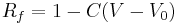$R_f=1-C(V-V_0)\,$
where:
Rf = frontal flow interception efficiency
C = constant, 0.09 - ENGLISH (0.295 - METRIC)
V = average velocity, ft/s (m/s)
V0 = splash-over velocity, ft/s (m/s)

The ratio of side flow intercepted to total side flow, Rs, can be determined using the equation: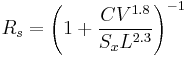$R_s=\left(1+\frac{CV^{1.8}}{S_xL^{2.3}}\right)^{-1}$
where:
Rs = side flow interception efficiency
C = constant, 0.15 - ENGLISH (0.0828 - METRIC)
V = average velocity, ft/s (m/s)
Sx = cross-slope adjacent to the grate (either pavement or gutter), ft/ft (m/m)
L = Length of grate, ft (m)

If the total flow (Q) is considered to be the sum of frontal flow (Qf) and side flow (Qs), the amount of flow intercepted by a grate inlet on grade (Qi) can be determined using the equation: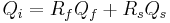$Q_i=R_fQ_f+R_sQ_s\,$

### 640.1.5.2 Capacity of Grate Inlets Located at Low Points in Grade

A grate type drop inlet located at a low point in grade will behave either as a weir or as an orifice, dependent upon the depth of water at the grate. The capacity is independent of the geometric configuration of the grate.

For depths of water less than 0.4 ft. (120 mm), the capacity is governed by weir flow and may be calculated by the following equation: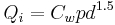$Q_i=C_wpd^{1.5}\,$
where:
Qi = inlet capacity, ft3/s (m3/s)
Cw = constant, 3.0 - ENGLISH (1.66 - METRIC)
p = effective perimeter of the grate, ft. (m)
d = depth of water above the top of the grate, ft. (m)

The effective perimeter can be calculated using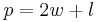$p=2w+l\,$ (when there is a curb)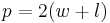$p=2(w+l)\,$ (when there is no curb)
where:
p = effective perimeter of the grate, ft. (m)
w = width of opening, ft. (m)
l = length of opening, ft. (m)

Only that portion of the grate's perimeter along which flow enters the inlet is considered when determining the effective perimeter.

When the depth of water exceeds 1.4 ft. (420 mm), the grate capacity is governed by orifice flow and may be calculated by the following equation: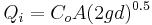$Q_i=C_oA(2gd)^{0.5}\,$
where:
Qi = inlet capacity, ft3/s (m3/s)
Co = orifice coefficient = 0.67
A = clear opening area of the grate, ft2 (m2)
g = 32.2 ft/s2 (9.80 m/s2)
d = depth of water above the top of the grate, ft. (m)

The clear opening area for MoDOT standard grates is given in the following table.

Clear Opening Area for MoDOT Standard Grates
Grate Type Clear Opening Area (A)
2 ft. x 2 ft. Parallel Bar Grate(610 mm x 610 mm) (with depressed cross bars) 3.04 ft2 (0.282 m2)
2 ft. x 3 ft. Parallel Bar Grate(610 mm x 914 mm) (with depressed cross bars) 4.56 ft2 (0.424 m2)
2 ft. x 4 ft. Parallel Bar Grate(610 mm x 1219 mm) (with depressed cross bars) 6.08 ft2 (0.565 m2)
2 ft. x 2 ft. Curved Vane Grate (610 mm x 610 mm) (bicycle safe) 2.10 ft2 (0.200 m2)
2 ft. x 4 ft. Curved Vane Grate (610 mm x 1219 mm) (bicycle safe) 4.20 ft2 (0.400 m2)
Parallel bar grates are not used for new installations. The clear opening areas are provided only for the purpose of analyzing existing inlets.

At depths between 0.4 and 1.4 ft. (120 and 420 mm), the grate operates in a transition zone. The actual capacity of the grate is less than that given by either the weir equation or the orifice equation. The capacity of the grate for depths within this transition zone can be estimated using a straight-line interpolation between the capacity at a depth of 0.4 ft. (120 mm) using the weir equation and the capacity at a depth of 1.4 ft. (420 mm) using the orifice equation.

Because of the uncertainty in the above equations, and because of the tendency for trash to clog the opening, the effective perimeter or the clear opening area of the grate should be at least twice that given by the above equations.

## 640.1.6 Capacity of Curb Opening Inlets

Like grate inlets, the capacity of curb opening inlets is a function of the inlet location. The intercept of curb opening inlets located on a continuous grade is a function of the length of the inlet, the amount the inlet is depressed, the total gutter flow and the depth of flow at the curb. The capacity of a curb opening inlet located at a low point is a function of the length of the opening and the depth of ponding.

### 640.1.6.1 Capacity of Curb Opening Inlets Located on a Continuous Grade

A curb opening inlet located on grade behaves as a falling head weir. In order to determine the capacity of such an inlet, it is first necessary to determine the length of curb opening required to intercept 100 percent of the gutter flow.

For a triangular pavement and gutter cross-section, the following equation is used to determine the length of curb opening required to intercept 100% of the gutter flow: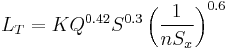$L_T=KQ^{0.42}S^{0.3}\left(\frac{1}{nS_x}\right)^{0.6}$
where:
LT = curb opening length required to intercept 100% of the gutter flow, ft. (m)
K = constant, 0.6 - ENGLISH (0.817 - METRIC)
Q = total gutter flow, ft3/s (m3/s)
S = longitudinal pavement slope (grade), ft/ft (m/m)
n = Manning's roughness coefficient (0.016 for gutters and pavement)
Sx = pavement and gutter cross-slope, ft/ft (m/m)

The efficiency of curb opening inlets shorter than the length required for 100% interception may be determined using the equation: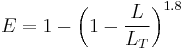$E=1-\left(1-\frac{L}{L_T}\right)^{1.8}$
where:
E = efficiency of the curb opening inlet
L = length of the curb opening, ft. (m)
LT = curb opening length required to intercept 100% of the gutter flow, ft. (m)

The inlet capacity can then be determined using the following equation: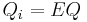$Q_i=EQ\,$
where:
Qi = the capacity of the curb opening inlet, ft3/s (m3/s)
E = the efficiency of the curb opening inlet
Q = total gutter flow, ft3/s (m3/s)

When a local depression is used to increase the capacity of the curb opening inlet, or when the pavement and gutter cross-slopes differ, an equivalent cross-slope (Se) must be used to determine the length required for 100% interception. The equivalent cross-slope may be found using the equation: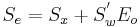$S_e=S_x+S_w^'E_o$
where:
Se = equivalent cross-slope, ft/ft [m/m]
Sx = normal pavement cross-slope, ft/ft [m/m]
S'w = cross-slope of the gutter section measured below the normal pavement cross-slope, ft/ft (m/m)
Eo = ratio of flow in the depressed section to total gutter flow, calculated just upstream of the inlet using the equations in Flow of Water in Gutters.

### 640.1.6.2 Capacity of Curb Opening Inlets Located at Low Points in Grade

Curb opening inlets at low points operate in either a submerged or an unsubmerged condition. When the depth of flow is less than approximately the height of the curb opening, the inlet is unsubmerged and operates as a weir. When the depth of flow is greater than approximately 1.4 times the height of the opening, the inlet is submerged and operates as an orifice.

The interception capacity for an unsubmerged curb opening inlet is determined using the following weir flow equation: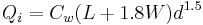$Q_i=C_w(L+1.8W)d^{1.5}\,$
where:
Qi = inlet capacity, ft3/s (m3/s)
Cw = constant, 2.3 - ENGLISH (1.25 - METRIC)
L = length of curb opening, ft. (m)
W = lateral width of depression, ft. (m)
d = depth at curb measured from the normal gutter or pavement cross-slope, ft. (m)

When the inlet is depressed, the weir location is at the lip of the depression, not at the lip of the curb opening. The weir length depends upon both the width of the depressed gutter and the length of the curb opening, and the weir equation is valid for depths at the curb up to approximately the height of the curb opening plus the depth of the depression.

The weir location for a curb opening inlet with no depression is at the lip of the opening and the weir length is equal to the length of the opening. For inlets without a local depression, the lateral width of depression can simply be set to zero in the above equation, and the equation is then valid for depths up to the height of the curb opening.

When the depth of flow is greater than 1.4 times the height of the curb opening, the opening is submerged. The capacity is then governed by orifice flow and may be calculated as follows: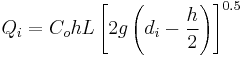$Q_i=C_ohL\left[2g\left(d_i-\frac{h}{2}\right)\right]^{0.5}$

where:
Qi = inlet capacity, ft3/s (m3/s)
Co = orifice coefficient = 0.67
h = height of curb opening, ft. (m)
L = length of curb opening, ft. (m)
g = 32.2 ft/s2 (9.80 m/s2)
di = depth of water at the lip of curb opening, ft. (m)

At depths between 1.0 and 1.4 times the height of the opening, the curb opening operates in a transition zone. The actual capacity of the curb opening is less than that given by either the weir equation or the orifice equation. The capacity of the curb opening for depths within this transition zone can be estimated using a straight-line interpolation between the capacity at a depth of 1.0 times the height of the opening, using the weir equation, and the capacity at a depth of 1.4 times the height of the opening, using the orifice equation.

## 640.1.7 Capacity of Combination Inlets

Combination inlets consist of a grate inlet and a curb inlet used at the same location. Considering combination inlets located on a continuous grade, the capacity of an unclogged combination inlet with the curb opening located adjacent to the grate may be assumed equal to the capacity of the grate inlet alone. Use of such inlets on a continuous grade is uneconomical except in areas where considerable debris may be expected. In this case, the curb opening provides a safety factor against clogging of the grate.

When the curb opening extends upstream from the grate, then the capacity of the inlet may be estimated as the capacity of that portion of the curb opening upstream of the grate, plus the capacity of the grate. The proper procedure for computing the capacity of such an inlet is to compute the intercept of the curb opening for that portion of the opening located upstream from the grate. This intercept is then subtracted from the total gutter flow, which yields the curb opening bypass. The grate inlet intercept is then computed using the curb inlet bypass as the approach gutter flow. The capacity of the combination inlet is the sum of the curb opening intercept and the grate intercept.

All debris carried by the storm water runoff that is not passed by upstream inlets must be passed by the inlet located at the low point. Therefore, these inlets are prone to clogging. As previously discussed, the capacity of a grate inlet at a low point may be computed by the proper equations. Also, it has been recommended that the computed effective perimeter, or clear waterway area, of the grate be doubled in order to provide a safety factor against clogging. However, if a combination inlet is used, the curb opening will provide the necessary safety factor against clogging, and the effective perimeter or clear area of the grate need only be equal to that given by the computations.